• Introduction
• What is in this manual
• What is Caspoc
• User interface
• Introduction
• Starting
• Simulation
• Editing
• Viewing and printing
• Getting Started
• Basic editing
• Simulation in the time domain
• Basic User Interface Topics
• Editing
• Simulation
• Viewing
• Library
• Reports
• Project management
• Circuit and Block Diagram Components
• Introduction
• Cscript and user defined functions
• Component parameters
• Modeling Topics
• Introduction
• Power Electronics
• Semiconductors
• Electrical Machines
• Electrical drives
• Power Systems
• Mechanical Systems
• Thermal Systems
• Magnetic Circuits
• Green Energy
• Coupling to FEM
• Experimenter
• Analog hardware description language
• Embedded C code Export
• Coupling to Spice
• Small Signal Analysis
• Matlab coupling
• Tips and tricks
• Appendices

## Basic editing.

Although explained in the "Getting Started" guide, we will give a brief resume of the basic operations in Caspoc

Both the circuit and the block-diagram are drawn in the same schematic. So your model contains the electric circuit, the control, the electrical load or electrical machine, plus an eventually mechanical system in one workspace.

The difference between the electric circuit and the block-diagram models is indicated by the appearance of the nodes.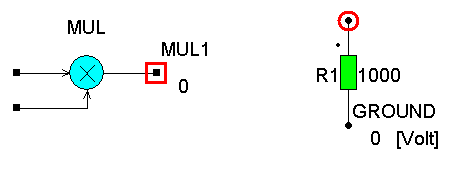Electric circuit

In the electric circuit you can model your power circuit and calculate voltages over components or between nodes and currents through components. The nodes are indicated by round dots.

One node should be assigned to be the reference ground node, which always has a voltage level of 0 volts. Caspoc automatically inserts a ground label, which you can replace afterwards. By clicking the node with the right-mouse button, a dialog box pops up where you can type the label of the node. For a ground reference label type "0" or "ground". To remove the label, press the [DEL] key.

Block-diagram

In the block-diagram you can model a dynamic system by using blocks which perform an operation on the inputs of the block. For example, an "ADD" block would add the signals at the two inputs and the result is available at the output. Block-diagram blocks are always operating from the inputs to the output and are automatically sorted by Caspoc. The nodes in the block-diagram are indicated by square dots.

Connection between the circuit and the block-diagram

You can measure voltages and currents from the electric circuit using the blocks "VOLTAGE" and "CURRENT" or one of the probes from the library.

Using the controlled voltage source "B" or controlled current source "A" a signal from the block diagram is used a current or voltage in the electric circuit.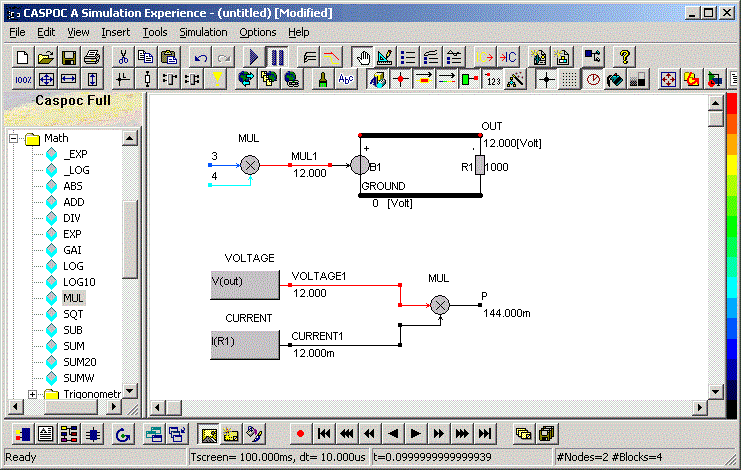Getting output

Use the "Scope" block to display any voltage, current, or block-diagram signal. You can insert a "Scope" in by clicking the first button on the bottom button bar.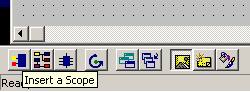And place the input of the "Scope" right on top of a circuit or block-diagram node. You can resize the "Scope" by clicking and dragging the right-bottom corner. The number of inputs automatically increases while resizing the "Scope".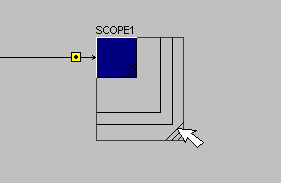To display a current through a circuit component, click with the left mouse button over the input of the "Scope", drag the mouse and release the left mouse button over the circuit component. A dashed line indicates the connection.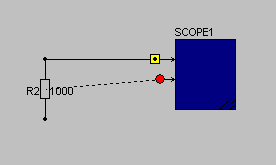"Scopes" are automatically scaling the results:

• at the end of the simulation
• when pressing the [F5] function key
• when continuing the simulation when pressing [ENTER]
Component values

You can assign values to components or edit parameters if you double- or right click the component with the mouse. For numerical values you can use the following abbreviations:

  T   = 1e12 G   = 1e9 MEG = 1e6 K   = 1e3 MIL = 25.4e-6   M = 1e-3 U = 1e-6 N = 1e-9 P = 1e-12 F = 1e-15 

For example, a 10000ohm resistor is written as 10k and a capacitor of 100 micro Farad as 100uF.

Simulation parameters

Two parameters have to be set to control the simulation. The parameter "Tscreen" defines the width of the "Scope" window. For displaying the simulation results in seconds.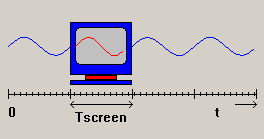The parameter "dT" defines the integration time step. As rule of thumb you can set it to approximately 1/10 to 1/00 of the reciprocal of the highest frequency occurring in your simulation. For example, use dT=100us and Tscreen=100ms for a line commutated converter operating a 50Hz or at 60Hz, or set dT=1us and Tscreen to 1ms for a Switched-Mode Power Supply operating at 10kHz.

Starting the simulation

Start the simulation by pressing the "Play" button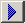or pressing the [Enter] key.

You can vary parameters during the simulation and simply continue by selecting the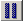pause/continue button, or pressing the [Enter] key.

Now that you understand the basic commands of the program, you are ready to start your own simulation projects.